# sklearn.model_selection.KFold¶

class sklearn.model_selection.KFold(n_splits='warn', shuffle=False, random_state=None)[source]

K-Folds cross-validator

Provides train/test indices to split data in train/test sets. Split dataset into k consecutive folds (without shuffling by default).

Each fold is then used once as a validation while the k - 1 remaining folds form the training set.

Read more in the User Guide.

Parameters: n_splits : int, default=3 Number of folds. Must be at least 2. Changed in version 0.20: n_splits default value will change from 3 to 5 in v0.22. shuffle : boolean, optional Whether to shuffle the data before splitting into batches. random_state : int, RandomState instance or None, optional, default=None If int, random_state is the seed used by the random number generator; If RandomState instance, random_state is the random number generator; If None, the random number generator is the RandomState instance used by np.random. Used when shuffle == True.

See also

StratifiedKFold
Takes group information into account to avoid building folds with imbalanced class distributions (for binary or multiclass classification tasks).
GroupKFold
K-fold iterator variant with non-overlapping groups.
RepeatedKFold
Repeats K-Fold n times.

Notes

The first n_samples % n_splits folds have size n_samples // n_splits + 1, other folds have size n_samples // n_splits, where n_samples is the number of samples.

Randomized CV splitters may return different results for each call of split. You can make the results identical by setting random_state to an integer.

Examples

>>> from sklearn.model_selection import KFold
>>> X = np.array([[1, 2], [3, 4], [1, 2], [3, 4]])
>>> y = np.array([1, 2, 3, 4])
>>> kf = KFold(n_splits=2)
>>> kf.get_n_splits(X)
2
>>> print(kf)
KFold(n_splits=2, random_state=None, shuffle=False)
>>> for train_index, test_index in kf.split(X):
...    print("TRAIN:", train_index, "TEST:", test_index)
...    X_train, X_test = X[train_index], X[test_index]
...    y_train, y_test = y[train_index], y[test_index]
TRAIN: [2 3] TEST: [0 1]
TRAIN: [0 1] TEST: [2 3]


Methods

 get_n_splits([X, y, groups]) Returns the number of splitting iterations in the cross-validator split(X[, y, groups]) Generate indices to split data into training and test set.
__init__(n_splits='warn', shuffle=False, random_state=None)[source]
get_n_splits(X=None, y=None, groups=None)[source]

Returns the number of splitting iterations in the cross-validator

Parameters: X : object Always ignored, exists for compatibility. y : object Always ignored, exists for compatibility. groups : object Always ignored, exists for compatibility. n_splits : int Returns the number of splitting iterations in the cross-validator.
split(X, y=None, groups=None)[source]

Generate indices to split data into training and test set.

Parameters: X : array-like, shape (n_samples, n_features) Training data, where n_samples is the number of samples and n_features is the number of features. y : array-like, shape (n_samples,) The target variable for supervised learning problems. groups : array-like, with shape (n_samples,), optional Group labels for the samples used while splitting the dataset into train/test set. train : ndarray The training set indices for that split. test : ndarray The testing set indices for that split.

## Examples using sklearn.model_selection.KFold¶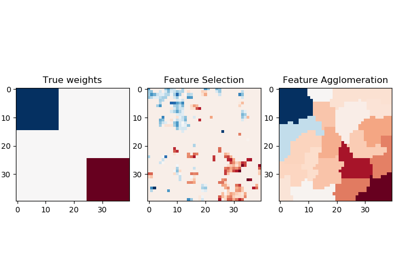Feature agglomeration vs. univariate selection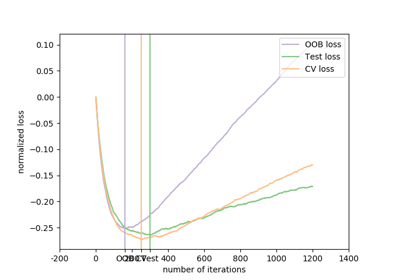Gradient Boosting Out-of-Bag estimates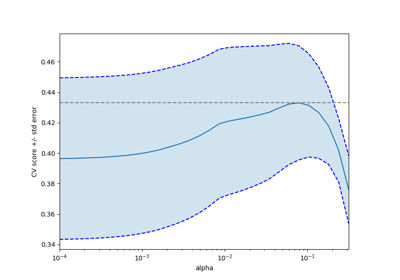Cross-validation on diabetes Dataset Exercise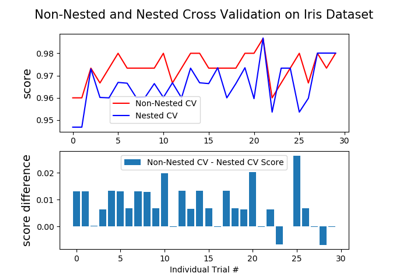Nested versus non-nested cross-validation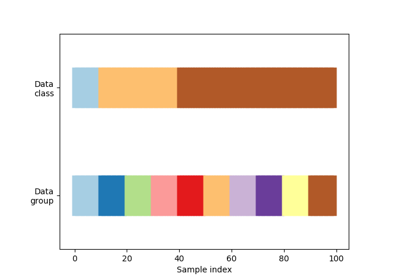Visualizing cross-validation behavior in scikit-learn